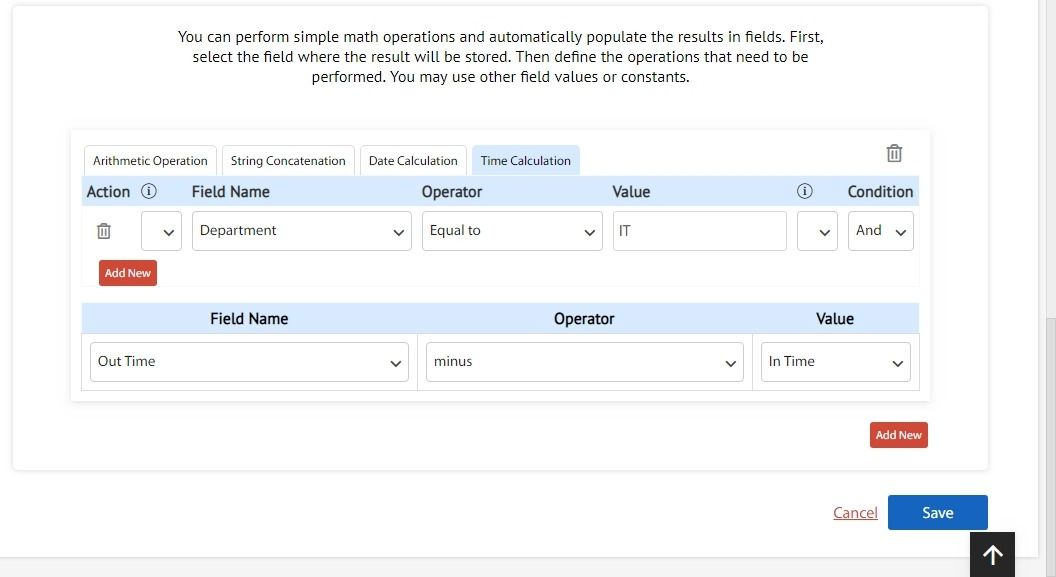Cflow provides a Time Calculation operation to perform the calculation based on time. You can perform simple math operation and automatically populate the result in a field. Use the Time field values to perform the calculation. In time calculation you can use only the operator minus to find the difference between the values.

For Example, In an organization, to know the total number of hours the employee has worked in a day. You can define the operations as shown in the picture below. When an employee enters the value in the In time and Out time field, automatically the number of hours is calculated and displayed in the Total number of hours field.